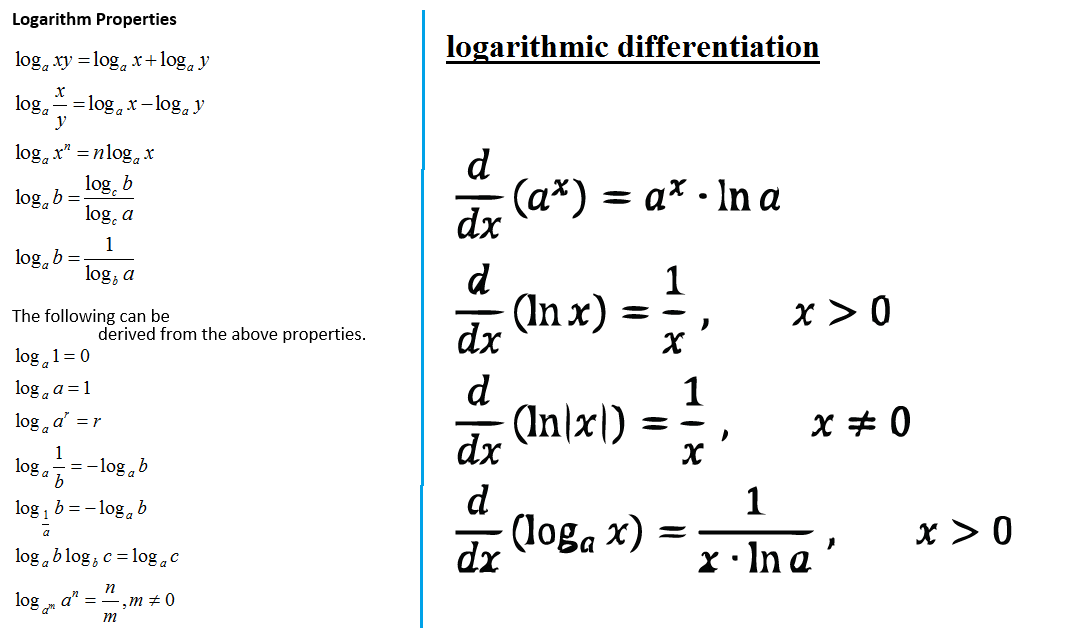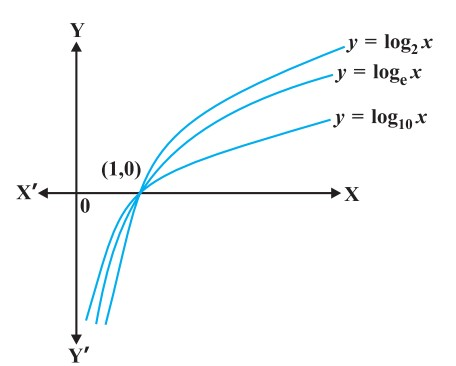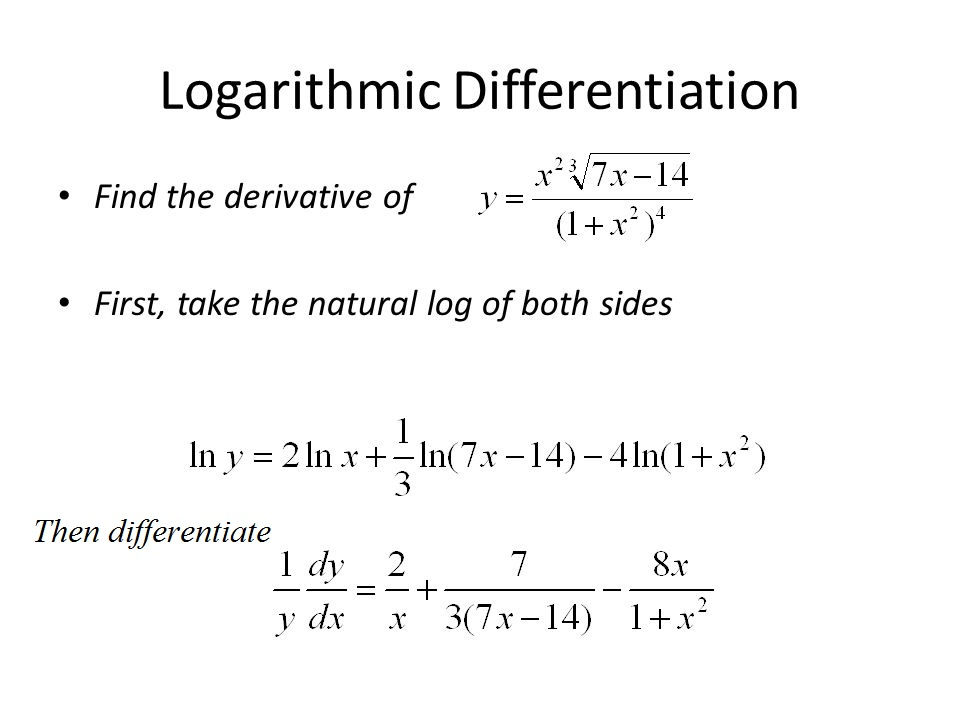Mathematics Exponential Functions,Logarithmic Functions And Logarithmic Differentiation For CBSE-NCERT
Click for Only Video

### Topic covered

star Exponential Functions
star Logarithmic Functions
star Logarithmic differentiation

### Exponential Functions● Definition : The exponential function is with positive base b > 1 is the function y = f(x) = b^x

● The graph of y = 10^x is given in the Fig

(1) Domain of the exponential function is R, the set of all real numbers.
(2) Range of the exponential function is the set of all positive real numbers.
(3) The point (0, 1) is always on the graph of the exponential function (this is a restatement of the fact that b^0= 1 for any real b > 1).
(4) Exponential function is ever increasing; i.e., as we move from left to right, the graph rises above.
(5) For very large negative values of x, the exponential function is very close to 0. In other words, in the second quadrant, the graph approaches x-axis (but never meets it).

=>color{orange}{"Exponential function with base 10 is called the common exponential function."}
color{orange}{"And series with base " e "instead of " 10 "This is called natural exponential function."}

Theorem :

1 . color{blue}{"The derivative of " \ \ a^x \ \ "w.r.t.," \ \x \ \ is \ \a^x.log_ea} ;

=>d/(dx) (a^x) =a^x log_ea

1 .color{blue}{"The derivative of " \ \ e^x \ \ "w.r.t.," \ \x \ \ is \ \e^x} ;

=>d/(dx) (e^x) =e^x
Q 3115723660Is it true that x = e^(log x) for all real x?
Class 12 Chapter 5 Example 28Solution:

First, observe that the domain of log function is set of all positive real numbers.
So the above equation is not true for non-positive real numbers. Now, let y = e^(log x). If
y > 0, we may take logarithm which gives us log y = log (e^(log x) ) = log x * log e = log x. Thus
y = x. Hence x = e^(log x) is true only for positive values of x.

One of the striking properties of the natural exponential function in differential
calculus is that it doesn’t change during the process of differentiation. This is captured
in the following theorem whose proof we skip.

### Logarithmic Functionscolor{red}{x → log_b x = y} if b^y = x

● This function, called the logarithmic function, is defined in log_b : R^+ → R

● As before if the base b = 10, we say it is common logarithms and if b = e, then we say it is natural logarithms.
Often natural logarithm is denoted by ln.Graph of log

Properties :

1. a^(log_(a) x) = x ; a ne 0 , ne 1 , x > 0

2. a^(log_(b) x ) = x^(log_b a) ; a , b > 0 , ne 1 , x > 0

3. log_a a =1, a > 0 , ne 1

4. log_a x = 1/(log_x a) ; x , a > 0 , ne 1

5. log_a x = (log_b x)/( log_b a) ; a , b > 0 , ne 1 , x > 0

6. For m, n > 0 and a > 0, ne 1, then

(i) log_a (m * n) = log_a m + log_a n

(ii) log_a (m/n) = log_a m - log_a n

(iii) log_a (m^n) = n log_a m

color{blue}{"Theorem"} : color{green}{"The derivative of log" \ \ x \ \ w.r.t., x\ \ i s\ \ 1/x}

=> d/(dx) (log x) =1/x
Q 3135723662Differentiate the following w.r.t. x:

(i) e^(-x)

(ii) sin (log x) , x > 0

(iii) cos^(-1) (e^x)

(iv) e^(cos x)

Class 12 Chapter 5 Example 29Solution:

(i) Let y = e^(– x). Using chain rule, we have

(dy)/(dx) = e^(-x) * d/(dx) (-x) = - e^(-x)

(ii) Let y = sin (log x) . Using chain rule, we have

(dy)/(dx) = cos (log x) *d/(dx ) (log x) = (cos (log x) )/x

(iii) Let y = cos^(–1) (e^x). Using chain rule, we have

(dy)/(dx) = (-1)/( sqrt ( 1- (e^x)^2 ) ) * d/(dx) (e^x) = (-e^x)/(sqrt (1- e^(2x) ) )

(iv) Let y = e^(cos x) . Using chain rule, we have

 (dy)/(dx) = e^(cos x) * (- sin x) = - ( sin x ) e^(cos x)

### Logarithmic Differentiation :● In this section, we will learn to differentiate certain special class of functions given in the form

=>y= f(x) =[ u(x)]^(v(x))

=> By taking logarithm (to base e) the above may be rewritten as

=> log y = v(x) log [u(x)]

=> Using chain rule we may differentiate this to get

1/y * (dy)/(dx) = v(x) * 1/(u(x)) * u'(x) + v'(x) * log [u(x)]

which implies that

color{orange}{(dy)/(dx) =y [(v(x))/(u(x)) * u'(x) + v'(x) * log[ u(x)]]}

Note :f (x) and u(x) must always be positive as otherwise their logarithms are not defined.
Q 3145723663Differentiate  sqrt ( ( (x-3) (x^2 +4) )/ ( 3x^2 + 4x +5) ) w.r.t. x.
Class 12 Chapter 5 Example 30Solution:

Let y = sqrt ( ( (x-3) (x^2 +4) )/( 3x^2 +4x +5) )

Taking logarithm on both sides, we have

log y = 1/2 [ log (x-3) + log (x^2 +4) -log (3x^2 +4x +5) ]

Now, differentiating both sides w.r.t. x, we get

1/y * (dy)/(dx) = 1/2 [ 1/(x-3) + (2x)/(x^2 +4) - (6x+4)/( 3x^2 +4x +5) ]

or (dy)/(dx) = y/2 [ 1/(x-3) + (2x)/(x^2 +4) - (6x+4)/( 3x^2 +4x +5) ]

=1/2 sqrt (( (x-3) (x^2 +4) )/( 3x^2 +4x +5) ) [1/(x-3) + (2x)/(x^2 +4) - (6x+4)/(3x^2 +4x + 5 ) ]
Q 3165723665Differentiate a^x w.r.t. x, where a is a positive constant.
Class 12 Chapter 5 Example 31Solution:

Let y = a^x. Then

log y = x log a

Differentiating both sides w.r.t. x, we have

1/y (dy)/(dx) = log a

or  (dy)/(dx ) = y log a

Thus  d/(dx) (a^x) = a^x log a
Q 3175723666Differentiate x^(sin x) , x > 0 w.r.t. x.
Class 12 Chapter 5 Example 32Solution:

Let y = x^(sin x) . Taking logarithm on both sides, we have

log y = sin x log x

Therefore  1/y * (dy)/(dx) = sin x d/(dx) (log x) + log x d/(dx) (sin x)

or 1/y (dy)/(dx) = (sin x) 1/x + log x cos x

or  (dy)/(dx ) = y [ (sin x)/x + cos x log x ]

= x^(sin x) [ (sin x)/x + cos x log x]

= x^(sin x-1) * sin x + x^(sin x) * cos x log x
Q 3185723667Find  (dy)/(dx)  , if y^x + x^y + x^x = a^b.
Class 12 Chapter 5 Example 33Solution:

Given that y^x + x^y + x^x = a^b.

Putting u = y^x, v = x^y and w = x^x, we get u + v + w = a^b

Therefore  (du)/(dx) + (dv)/(dx) + (dw)/(dx) = 0 ........(1)

Now, u = y^x. Taking logarithm on both sides, we have

log u = x log y

Differentiating both sides w.r.t. x, we have

1/u * (du)/(dx) = x d/(dx) (log y ) + log y d/(dx) (x)

= x 1/y * (dy)/(dx) + log y * 1

so (du)/(dx) = u (x/y (dy)/(dx) + log y) = y^x [x/y (dy)/(dx) + log y ] .... (2)

Also v = x^y

Taking logarithm on both sides, we have

log v = y log x

Differentiating both sides w.r.t. x, we have

1/v * (dv)/(dx) = y d/(dx) (log x) + log x (dy)/(dx)

 = y * 1/x + log x * (dy)/(dx)

So  (dv)/(dx) = v [ y/x + log x (dy)/(dx) ]

 = x^y [y/x + log x (dy)/(dx) ] ................(3)

Again w = x^x

Taking logarithm on both sides, we have

log w = x log x.

Differentiating both sides w.r.t. x, we have

1/w* (dw)/(dx) = x d/(dx) (log x) + log x * d/(dx) (x)

= x * 1/x + log x * 1

i.e.  (dw)/( dx) = w (1+ log x)

= x^x (1+ log x) ...................(4)

From (1), (2), (3), (4), we have

y^x ( x/y (dy)/(dx) + log y) + x^y (y/x + log x (dy)/(dx) ) + x^x (1+ log x) = 0

or  ( x * y^(x-1) + x^y * log x) (dy)/(dx) = - x^x (1+ log x ) - y * x^(y-1) - y^x log y

Therefore  (dy)/(dx) = ( - [ y^x log y + y * x^(y-1) + x^x (1+ log x) ] )/( x * y^(x-1) + x^y log x)